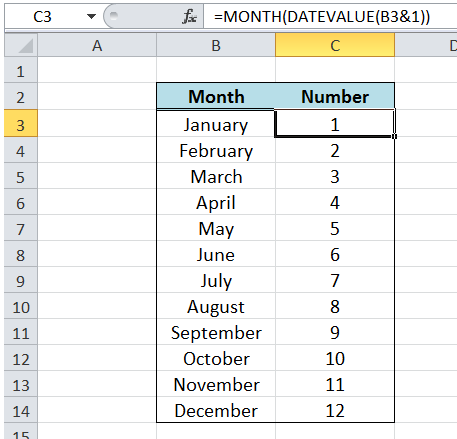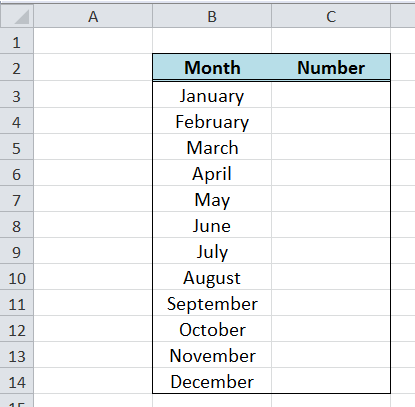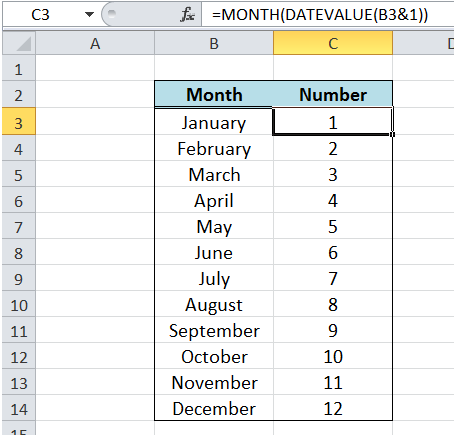Get instant live expert help with Excel or Google Sheets“My Excelchat expert helped me in less than 20 minutes, saving me what would have been 5 hours of work!”

#### Post your problem and you'll get expert help in seconds

Your message must be at least 40 characters
Our professional experts are available now. Your privacy is guaranteed.

# Convert Month Name to Number in Excel

Excel enables us to convert month name to a number, by using the two functions MONTH and DATEVALUE.Figure 1.  Final result:  Convert Month Name to Number

## Syntax of MONTH function

`=MONTH(serial_number)`

• serial_number – the date whose month we are trying to find; Dates should be entered in a date format and not as text

## Syntax of DATEVALUE function

DATEVALUE function converts a date stored as text into a serial number, where the value 1 represents the date Jan 1, 1900 or 1/1/1900, and an increment of 1 means one day thereafter

`=DATEVALUE(date_text)`

• Date_text – a text string representing a date in Excel
• If the year portion in the date is omitted, the current year is used by the function
• The date must be between January 1, 1900 and December 31, 9999, otherwise the DATEVALUE function returns the error #VALUE!

## Convert month name to number

Suppose we have a list of month names that we want to convert into a number.  It could be done manually wherein January is 1, February is 2, and so on up to December which is 12.

Fortunately, Excel provides us with a formula that can automatically convert month name to number, using the two functions MONTH and DATEVALUE.Figure  2. Sample list of month names to be converted to number

## Formula to convert month name to number

`=MONTH(DATEVALUE(B3&1))  `

The MONTH function returns the month of any date in its argument. The argument is the date returned by the DATEVALUE function.   In Excel, month names alone cannot be used in formulas.  In this case, DATEVALUE is able to interpret the month name linked to the number 1 as a date.

### Example:

DATEVALUE(B3&1) is interpreted as DATEVALUE(January1)

## How to convert month to number?

Step 1.  Enter the formula  =MONTH(DATEVALUE(B3&1))   in cell C3

Step 2.  Copy the formula and paste to the other cells C4:C14Figure 3. Using MONTH and DATEVALUE to convert month name to number

Finally, we have converted the month names in column B to corresponding numbers in column D.

## Instant Connection to an Expert through our Excelchat Service

Most of the time, the problem you will need to solve will be more complex than a simple application of a formula or function. If you want to save hours of research and frustration, try our live Excelchat service! Our Excel Experts are available 24/7 to answer any Excel question you may have. We guarantee a connection within 30 seconds and a customized solution within 20 minutes.